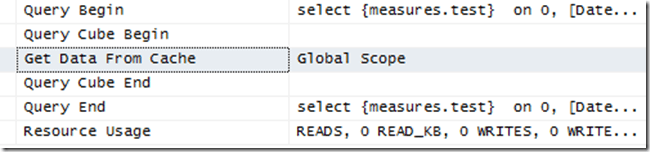# SSAS Multidimensional Formula Engine Caching and Locale-Dependent Properties

One subject I have blogged about many times here is how the use of certain MDX functions and features prevents the Formula Engine from caching the result of a calculation for longer than the lifetime of a query (see here and here for just two examples). Reading the new SSAS 2012/2014 Performance Guide, I spotted the following:

The use of MDX functions that are locale-dependent (such as CAPTION or .Properties) prevents the use of the global cache, because different sessions may be connected with different locales and cached results for one locale may not be correct for another locale.

It’s a bit vague but here’s an example of it happening. Take the following calculated measure for the Adventure Works cube:

`CREATE MEMBER CURRENTCUBE.MEASURES.TEST AS`

`IIF(`

`[Date].[Date].CURRENTMEMBER.PROPERTIES("MEMBER_NAME")="July 1, 2001",`

`[Measures].[Internet Sales Amount],`

`[Measures].[Internet Tax Amount]);`

If you run the following query, first on a cold cache and then on a warm cache:

`select`

`{measures.test} `

`on 0,`

`[Date].[Date].[Date].members`

`on 1`

`from`

`[Adventure Works]`

In Profiler you will see that the second time it is run, the values for the calculated measure are returning from the Formula Engine cache:Here, the Get Data From Cache event is showing that on the second execution of the query the Formula Engine cache is being used.

However, if you change the calculation so it uses the MEMBER_CAPTION property instead of the MEMBER_NAME property, like so:

`CREATE MEMBER CURRENTCUBE.MEASURES.TEST AS`

`IIF(`

`[Date].[Date].CURRENTMEMBER.PROPERTIES("MEMBER_CAPTION")="July 1, 2001",`

`[Measures].[Internet Sales Amount],`

`[Measures].[Internet Tax Amount]);`

Then run the query again, on a cold cache then a warm cache, you will see the following:Here the Storage Engine cache is being used – and since the query only contains a single calculated measure, this means that the Formula Engine cache is not being used, and may result in noticeably worse overall query performance if your calculation is expensive.

The reason there is a difference between MEMBER_NAME and MEMBER_CAPTION is that the former property will return the same value for all users, but the latter may return different values for users in different locales. Therefore it is not safe to cache the results of calculations that use the MEMBER_CAPTION property because these calculations could return different values for different users for the same query.